# Quadratic equations - how do you.....

1. find the quadratic equation when you are only given the graph of the parabola and from the graph, you can interpret 2 x-intercepts  (0.5,0) (-2,0) and the vertex (-0.5,-2)?

This question was given at a Grade 10 level with only the basic understanding of quadratics and polynomials - standard form and binomials.

Help?

1.0
sandra rinckposted 12 years agoin reply to this

I have really no idea but trying to visual this in my head, it "seems" like it would be were the two linear lines intersect on a graph heading in opposite directions.  I really don't know though.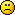2. This is an atrocious question ... posed by a person who, from her logo, appears to be of a radically different kind ...

Besides, I am not familiar with this Applied Physics jargon-ism and Semantics ... I pray ... for Understanding.

Anyway, if you could ask this question with Graphic Depiction of the Postulated ... I may be able to decipher, and give you an answer.

1. This is an applied mathematical question -- how my avatar looks has no bearing on the question nor is this an "atrocious" question. I don't see the relationship between these opinions on the question.

It is a simple question -- graph the parabola with the x-intercepts and the vertex I have given.  I can't sketch one on the forum.

If you like to help me out, then thank you but I could do without the negativity and judgemental attitude.

1. I can now "see"  ... your limitations.

But try remember, the one you see, in the mirror, everyday ... is a total stranger, to me ...

And I am not trying being judgemental, or negative ... I am simply stating the Truth.

1. I'm glad you can see everyone else's limitations.  Can you see your own?  I hope you can, otherwise, you've limited yourself.

As for truth, truth is only how you perceive and interpret it.  There are many facets to truth and many more ways to interpret those facets.  Perhaps you should watch the movie "What the Bleep" as well as "Down the Rabbit Hole".  If you do, you would understand what I am saying.

You're version of truth may not necessarily be mine or anyone elses for I am only in your truth because you wish that I am a part of your truth.  You are in my version of truth because I wish for you to be here for my own reasons.  Limitations are set only by closed minds and those minds who believe they have found what they seek.

Now, my OP was strictly a request for assistance with a mathematical question.  This is NOT a religious thread nor was it placed in the religious forum or in the philosophical forum.

I'd appreciate that any more insuations you make towards religious belief or otherwise be kept out of my thread.  Unless you have a response to my original mathematical question, please don't post anymore.  I am not interested in a religious or philosophical debate.  I'm here for an answer regarding a MATHEMATICAL question.  Got it?

Thank you.

3. I'm just a math teacher so I will just give a plain answer.
You have a parabola with x-intercepts (0.5,0) and (-2,0).I wish I had software to show you a sketch of this. By the properties of Parabolas (Symmetric with respect to line passing through vertex), the vertex should be on the vertical line passing through the midpoint of (0.5, 0) and (-2,0). This midpoint is at (-0.75,0) not  (-0.5, 0). However it is often difficult to read points on graphs with too small a scale.The vertex form of a quadratic is y = a(x-h)^2 +k . (h,k) being the vertex. From the data given:
y= a(x+0.75)^2 -2.We still need to calculate a.Substituting
the intercept (-2,0) yields 0=a(-2+0.75)^2 -2 . Solving for a
a = 2/ (-1.25)^2
a = 1.28

4. Assuming (-0.5,-2) is not the vertex but just another point on the quadratic.
The equation for a quadratic is y = ax^2 +bx +c
Substitute the coordinates of the points (-0.5,-2), (0.5,0) and (-2,0) to obtain:
-2 = a (-0.5)^2 + b(-0.5) + c    or -2 = 0.25a -o.5b +c
0 = a(0.5)^2 +b(0.5) +c or 0 =0.25a +0.5b +c
and
0 = a(-2)^2 + b(-2) +c or 4a -2b+c =0.
Now solve for a, b, and c.Unfortunately one obtains an impossible answer . So I think my previous post is probably more correct

2. Thanks for trying to help out Sandra.  At least you tried.... It's a parabola that turns upwards for inifity.  The vertex doesn't sit on the y axis...  this makes it a bit more difficult, especially when I cannot teach this using a higher level of calculus.  I'm stuck trying to teach this at a Grade 10 level.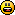1. Beth I think y= x squared +3/2x -1 works. (sorry don't know how to do maths symbols on computer. In words y=x squared +  one and a half x -1.
(0.5, 0) 0.5sq =1/4
+3/2x          =3/4
-1              =0

(-2, 0)
-2 sq      =4
+3/2x      =-3
-1          =0

I think this is right. So x squared +3/2x -1 = 0

3. Seanorjohn,  I think you're right too.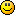But, if y=ax^2 + bx + c,

then how did you arrive at +3/2x?

I can't relate the numbers to their positions.  I've tried working backwards, but only to arrive at frustration.Can you explain the +3/2x?

Thank you for your help.  You're always there....and I never know that you are.

How are you??4. Hello I am Sandra,

I guess you will not surprise to receive my mail? i saw your profile

and it
sound well.I will like us to exchange good relationship.I am Sandra
by name,No kid and never marry.from here you can contact me at this
email address (sandrakelvin94@yahoo.com )) so that i can send you my
pictures and also tell you more about myself,have a nice day
and i am waiting to hear from you snoonest,

Remeber the distance or colour does not matter but love and concern

matters alot in life

(sandrakelvin94 at yahoo dut com )
Yours
Sandra

5. Beth, Go from basics.
y=a*x^2+b*x+c is the function for the parabola. you have thre points on the parabola.
replacing x and y you get three equations that are easy to solve as the intercepts are given.
the three equations are
4*a-2*b+c=0
0.25*a+0.5*b+c=0
0.25*a-0.5*b+c=-2

6. Hi Beth I am fine thanks.I have a fondness for all things canadian, so I notice your posts.

How I got to 3/2 x. Well, some call it:
trial and error
intuition
lucky guess

I didn't get to it in the way a real mathematician would. So I don't think I can really help.

A very quick sketch graph shows the graph will pass through the y intercept about -1.
Then I looked at the simplest set of co-ordinates and did 0.5^2 and realised I would need to get anther 3/4. So I noticed 1.5 x 0.5 would get me it.

Apologies for such a rubbish explanation. I guess I just got lucky.

Noticed two rather strange replies to this topic. Sounds like Sandra has fallen in love with you Beth. Who would have thought quadratic equations could lead to such passion.

7. You need the shape vertex formula

Replace h; k in the Shape-Vertex Formula
f(x) = a(x - h)^2 + k;

h= -0.5
k= -2

now
y=a(x-(-0.5)^2 + (-2)
y= a(x+0.5)^2 - 2

Here's with (0.5,0)
y= a(x+0.5)^2 - 2

0= a(0.5+0.5)^2 - 2
a=2

y=2(x+0.5)^2 - 2
y=2(x^2+1x+.25) - 2
y=2x^2+2x-1.5

or

y=ax^2 + bx + C

8. @ Sean -- (oh yea, remember I said I would call you Sean?) ha ha  thanks for your explanation.  I knew you had some logic in it.  As for Sandra, well, maybe she was speaking to you?I wouldn't blame her.

@ sunpen -- ah, I see.  Thank you.  I was stuck after replacing x and y intercepts.  I think I have it now.@ ediggity -- I tried it originally, but got all confused.  lol  with your explaination, I see where I went wrong.  I had h and k mixed up -- I can structure building to not fall down, but I can't solve a simple math problem!!! go figure!!  ha ha ha  Thanks ediggity -- I really appreciate the step by step -- it's exactly what I needed!1.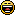hello Beth, i just passed these math couple of years ago. And I knew the answer.

people love you.are you a teacher?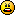9. This is impossible given the parameters of those three points.  The vertex always lies half way between the two x intercepts.  Given your intercepts, you would get the equation:
y=2x^2+3x-2

Using ediggity's equation, you would get the right vertex and one intercept, but not the other.

In the screenshot, my eq is in gray, ediggity's is in black.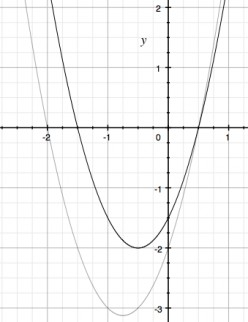1. Funnily enough I thought the same. I conveniently ignored the third thingyme jig.

teach us the right way. Give us an example . And thanks.

2. Ahh good catch.  I didn't bother to graph, or use the other two points to check the Vertex Formula.

3. Your graph's defined differential, is essentially theoreical, thus, a biased representation of The Law ...

Therefore, your Graph is just "one" ... of the countless permutation possibilities ... hence, not representing ... The Truth ...

The assumed is the state of a false postulate.

goodbye

10. Beth, you are getting sensible answers but also some weird answers.
By the way, Sandra was def after you.

11. This question is a bit tricky.  It is hard to pinpoint this because we are not used to writing equations based on x intercept equations.

If you have 2 points you can express a quadratic equation.  It is nice that we were given the zeros or intercepts.

1st:  (0.5,0) (-2,0) gives you where the zeros are in an equation.

So this equation becomes:

yX-.5)(X+2) which is y=X^2 +1.5X -1

Lets test and see if the vertex is true for this equation.

vertex is the middle of .5 and -2.

There is an error the vertex should be at -.75 not at -.5.  This is what is making this harder.

However based on just the two points, you can write an equation.

I hope this helps.

1. How did this put a sad face in my equation.

y = (x-.5)(x+2)

12. @ saleheen --you're too sweet!

@ doster -- ah!  I got it!!!  You're right...the two intercepts will not produce the vertex.  I'll go back and check the graph more closely.  I had to interpret it myself, and in the darkness (middle of the night) I might have read it wrong.  Plus, I was too frustrated.  I'll get back on the vertex....

@ sean -- ha ha  perhaps we can start one together!(I still think she was hitting on you!)

@ ediggity -- yes, good catch by doster.  But, you've explained it plainly so I could understand.@ ben -- yea, I was wondering how the sad face ended up in there too...unless you saw how I looked when I couldn't figure the question out!did you know, I tried to find an example out there via google, bing, yahoo and every other search engine only to NOT find an example?!?

I'm very grateful for all the help!!!I will get back on the vertex (or the x-intercept that is incorrect) so that I can get this question squared away.1. I'd like to see your vertex!13. I know nothing about math - never seen a vertex!  but definitely Sandra was just one of those random posters looking for love in all the wrong places.14. @ logic -- ha ha  I bet you would!@ mega -- it's been sooo long!  how are you?  Yea, sandra's looking for love in the wrong thread!  but, I'll send her some hub-love!15. @ habmath -- yes, I made a mistake reading the graph.  It is -0.75 and not -0.5.

Thank you for explaining it so simply.  Now that I understand what h and k represents, this will be easy to teach this to the students.*light bulb goes on brightly*working